# Category: number theory

Dedekind’s Psi-function $\Psi(n)= n \prod_{p |n}(1 + \frac{1}{p})$ pops up in a number of topics:

• $\Psi(n)$ is the index of the congruence subgroup $\Gamma_0(n)$ in the modular group $\Gamma=PSL_2(\mathbb{Z})$,
• $\Psi(n)$ is the number of points in the projective line $\mathbb{P}^1(\mathbb{Z}/n\mathbb{Z})$,
• $\Psi(n)$ is the number of classes of $2$-dimensional lattices $L_{M \frac{g}{h}}$ at hyperdistance $n$ in Conway’s big picture from the standard lattice $L_1$,
• $\Psi(n)$ is the number of admissible maximal commuting sets of operators in the Pauli group of a single qudit.

The first and third interpretation have obvious connections with Monstrous Moonshine.

Conway’s big picture originated from the desire to better understand the Moonshine groups, and Ogg’s Jack Daniels problem
asks for a conceptual interpretation of the fact that the prime numbers such that $\Gamma_0(p)^+$ is a genus zero group are exactly the prime divisors of the order of the Monster simple group.

Here’s a nice talk by Ken Ono : Can’t you just feel the Moonshine?

For this reason it might be worthwhile to make the connection between these two concepts and the number of points of $\mathbb{P}^1(\mathbb{Z}/n\mathbb{Z})$ as explicit as possible.

Surely all of this is classical, but it is nicely summarised in the paper by Tatitscheff, He and McKay “Cusps, congruence groups and monstrous dessins”.

The ‘monstrous dessins’ from their title refers to the fact that the lattices $L_{M \frac{g}{h}}$ at hyperdistance $n$ from $L_1$ are permuted by the action of the modular groups and so determine a Grothendieck’s dessin d’enfant. In this paper they describe the dessins corresponding to the $15$ genus zero congruence subgroups $\Gamma_0(n)$, that is when $n=1,2,3,4,5,6,7,8,9,10,12,13,16,18$ or $25$.

Here’s the ‘monstrous dessin’ for $\Gamma_0(6)$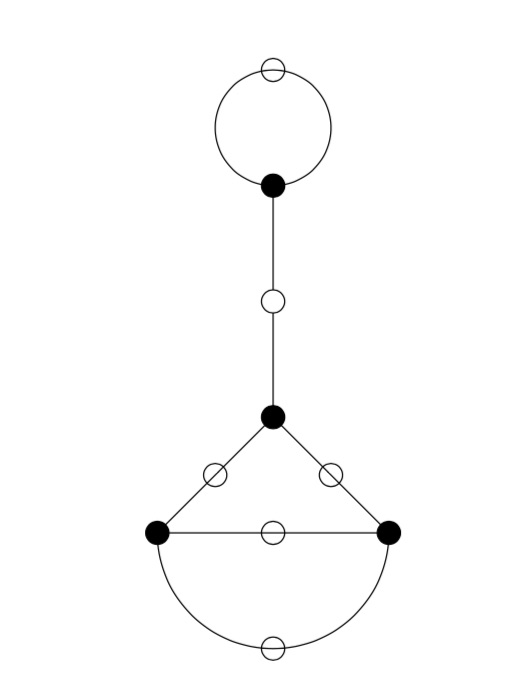But, one can compute these dessins for arbitrary $n$, describing the ripples in Conway’s big picture, and try to figure out whether they are consistent with the Riemann hypothesis.

We will get there eventually, but let’s start at an easy pace and try to describe the points of the projective line $\mathbb{P}^1(\mathbb{Z}/n \mathbb{Z})$.

Over a field $k$ the points of $\mathbb{P}^1(k)$ correspond to the lines through the origin in the affine plane $\mathbb{A}^2(k)$ and they can represented by projective coordinates $[a:b]$ which are equivalence classes of couples $(a,b) \in k^2- \{ (0,0) \}$ under scalar multiplication with non-zero elements in $k$, so with points $[a:1]$ for all $a \in k$ together with the point at infinity $[1:0]$. When $n=p$ is a prime number we have $\# \mathbb{P}^1(\mathbb{Z}/p\mathbb{Z}) = p+1$. Here are the $8$ lines through the origin in $\mathbb{A}^2(\mathbb{Z}/7\mathbb{Z})$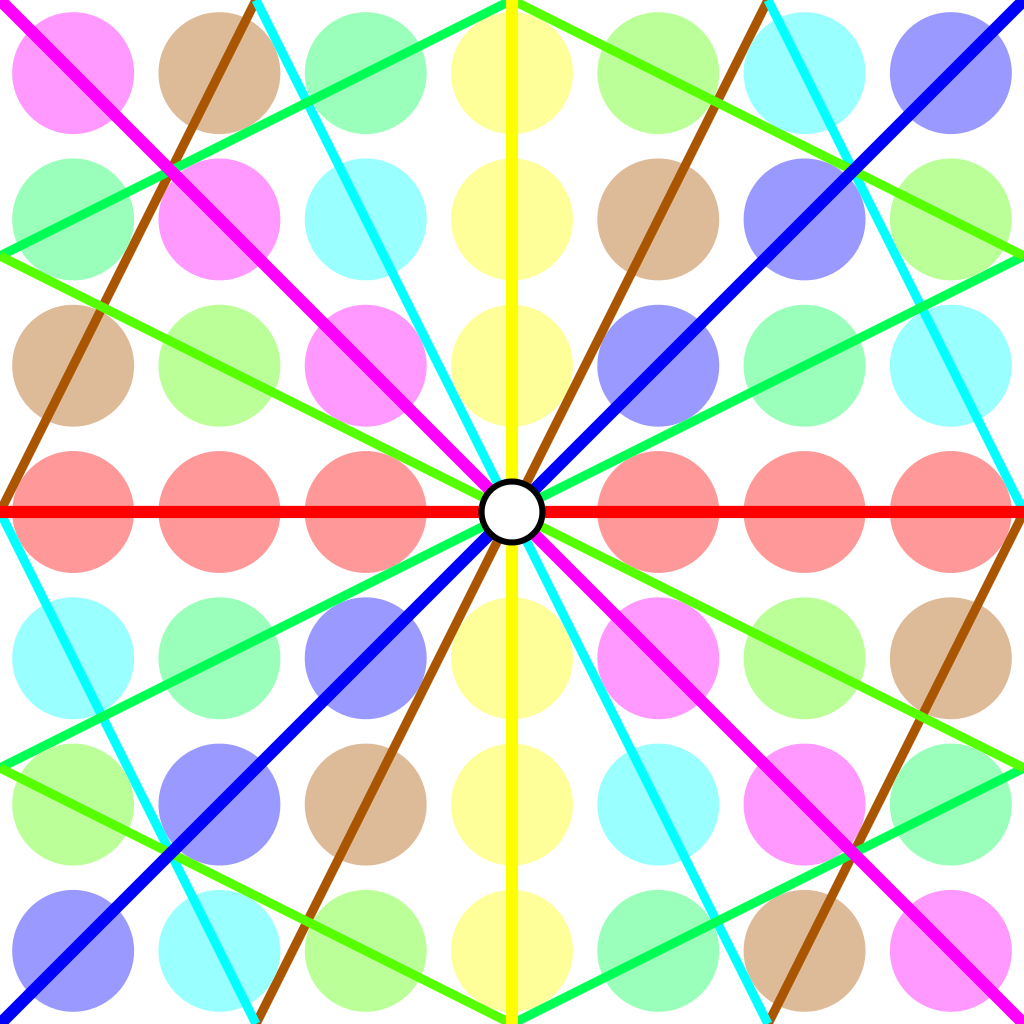Over an arbitrary (commutative) ring $R$ the points of $\mathbb{P}^1(R)$ again represent equivalence classes, this time of pairs
$(a,b) \in R^2~:~aR+bR=R$
with respect to scalar multiplication by units in $R$, that is
$(a,b) \sim (c,d)~\quad~\text{iff}~\qquad \exists \lambda \in R^*~:~a=\lambda c, b = \lambda d$
For $\mathbb{P}^1(\mathbb{Z}/n \mathbb{Z})$ we have to find all pairs of integers $(a,b) \in \mathbb{Z}^2$ with $0 \leq a,b < n$ with $gcd(a,b)=1$ and use Cremona’s trick to test for equivalence:
$(a,b) = (c,d) \in \mathbb{P}^1(\mathbb{Z}/n \mathbb{Z})~\quad \text{iff}~\quad ad-bc \equiv 0~mod~n$
The problem is to find a canonical representative in each class in an efficient way because this is used a huge number of times in working with modular symbols.

Perhaps the best algorithm, for large $n$, is sketched in pages 145-146 of Bill Stein’s Modular forms: a computational approach.

For small $n$ the algorithm in $\S 1.3$ in the Tatitscheff, He and McKay paper suffices:

• Consider the action of $(\mathbb{Z}/n\mathbb{Z})^*$ on $\{ 0,1,…,n-1 \}=\mathbb{Z}/n\mathbb{Z}$ and let $D$ be the set of the smallest elements in each orbit,
• For each $d \in D$ compute the stabilizer subgroup $G_d$ for this action and let $C_d$ be the set of smallest elements in each $G_d$-orbit on the set of all elements in $\mathbb{Z}/n \mathbb{Z}$ coprime with $d$,
• Then $\mathbb{P}^1(\mathbb{Z}/n\mathbb{Z})= \{ [c:d]~|~d \in D, c \in C_d \}$.

Let’s work this out for $n=12$ which will be our running example (the smallest non-squarefree non-primepower):

• $(\mathbb{Z}/12\mathbb{Z})^* = \{ 1,5,7,11 \} \simeq C_2 \times C_2$,
• The orbits on $\{ 0,1,…,11 \}$ are
$\{ 0 \}, \{ 1,5,7,11 \}, \{ 2,10 \}, \{ 3,9 \}, \{ 4,8 \}, \{ 6 \}$
and $D=\{ 0,1,2,3,4,6 \}$,
• $G_0 = C_2 \times C_2$, $G_1 = \{ 1 \}$, $G_2 = \{ 1,7 \}$, $G_3 = \{ 1,5 \}$, $G_4=\{ 1,7 \}$ and $G_6=C_2 \times C_2$,
• $1$ is the only number coprime with $0$, giving us $[1:0]$,
• $\{ 0,1,…,11 \}$ are all coprime with $1$, and we have trivial stabilizer, giving us the points $[0:1],[1:1],…,[11:1]$,
• $\{ 1,3,5,7,9,11 \}$ are coprime with $2$ and under the action of $\{ 1,7 \}$ they split into the orbits
$\{ 1,7 \},~\{ 3,9 \},~\{ 5,11 \}$
giving us the points $[1:2],[3:2]$ and $[5:2]$,
• $\{ 1,2,4,5,7,8,10,11 \}$ are coprime with $3$, the action of $\{ 1,5 \}$ gives us the orbits
$\{ 1,5 \},~\{ 2,10 \},~\{ 4,8 \},~\{ 7,11 \}$
and additional points $[1:3],[2:3],[4:3]$ and $[7:3]$,
• $\{ 1,3,5,7,9,11 \}$ are coprime with $4$ and under the action of $\{ 1,7 \}$ we get orbits
$\{ 1,7 \},~\{ 3,9 \},~\{ 5,11 \}$
and points $[1:4],[3:4]$ and $[5,4]$,
• Finally, $\{ 1,5,7,11 \}$ are the only coprimes with $6$ and they form a single orbit under $C_2 \times C_2$ giving us just one additional point $[1:6]$.

This gives us all $24= \Psi(12)$ points of $\mathbb{P}^1(\mathbb{Z}/12 \mathbb{Z})$ (strangely, op page 43 of the T-H-M paper they use different representants).

One way to see that $\# \mathbb{P}^1(\mathbb{Z}/n \mathbb{Z}) = \Psi(n)$ comes from a consequence of the Chinese Remainder Theorem that for the prime factorization $n = p_1^{e_1} … p_k^{e_k}$ we have
$\mathbb{P}^1(\mathbb{Z}/n \mathbb{Z}) = \mathbb{P}^1(\mathbb{Z}/p_1^{e_1} \mathbb{Z}) \times … \times \mathbb{P}^1(\mathbb{Z}/p_k^{e_k} \mathbb{Z})$
and for a prime power $p^k$ we have canonical representants for $\mathbb{P}^1(\mathbb{Z}/p^k \mathbb{Z})$
$[a:1]~\text{for}~a=0,1,…,p^k-1~\quad \text{and} \quad [1:b]~\text{for}~b=0,p,2p,3p,…,p^k-p$
which shows that $\# \mathbb{P}^1(\mathbb{Z}/p^k \mathbb{Z}) = (p+1)p^{k-1}= \Psi(p^k)$.

Next time, we’ll connect $\mathbb{P}^1(\mathbb{Z}/n \mathbb{Z})$ to Conway’s big picture and the congruence subgroup $\Gamma_0(n)$.

Last time we revisited Robin’s theorem saying that 5040 being the largest counterexample to the bound
$\frac{\sigma(n)}{n~log(log(n))} < e^{\gamma} = 1.78107...$ is equivalent to the Riemann hypothesis.

There’s an industry of similar results using other arithmetic functions. Today, we’ll focus on Dedekind’s Psi function
$\Psi(n) = n \prod_{p | n}(1 + \frac{1}{p})$
where $p$ runs over the prime divisors of $n$. It is series A001615 in the online encyclopedia of integer sequences and it starts off with

1, 3, 4, 6, 6, 12, 8, 12, 12, 18, 12, 24, 14, 24, 24, 24, 18, 36, 20, 36, 32, 36, 24, 48, 30, 42, 36, 48, 30, 72, 32, 48, 48, 54, 48, …

and here’s a plot of its first 1000 values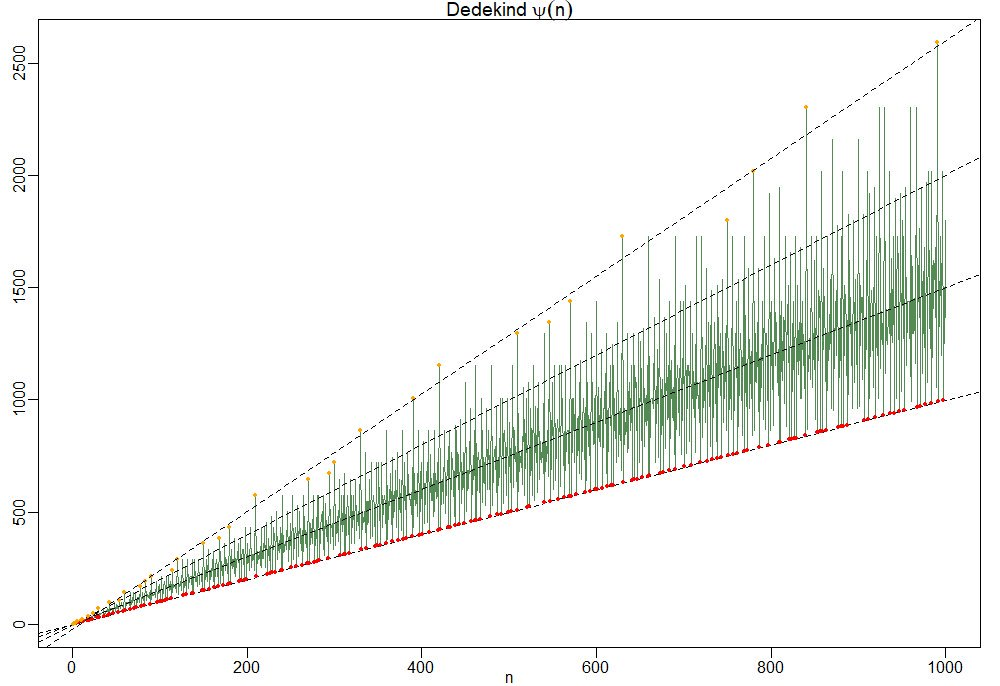To understand this behaviour it is best to focus on the ‘slopes’ $\frac{\Psi(n)}{n}=\prod_{p|n}(1+\frac{1}{p})$.

So, the red dots of minimal ‘slope’ $\approx 1$ correspond to the prime numbers, and the ‘outliers’ have a maximal number of distinct small prime divisors. Look at $210 = 2 \times 3 \times 5 \times 7$ and its multiples $420,630$ and $840$ in the picture.

For this reason the primorial numbers, which are the products of the fist $k$ prime numbers, play a special role. This is series A002110 starting off with

1, 2, 6, 30, 210, 2310, 30030, 510510, 9699690, 223092870,…

In Patrick Solé and Michel Planat Extreme values of the Dedekind $\Psi$ function, it is shown that the primorials play a similar role for Dedekind’s Psi as the superabundant numbers play for the sum-of-divisors function $\sigma(n)$.

That is, if $N_k$ is the $k$-th primorial, then for all $n < N_k$ we have that the 'slope' at $n$ is strictly below that of $N_k$ $\frac{\Psi(n)}{n} < \frac{\Psi(N_k)}{N_k}$ which follows immediately from the fact that any $n < N_k$ can have at most $k-1$ distinct prime factors and $p \mapsto 1 + \frac{1}{p}$ is a strictly decreasing function.

Another easy, but nice, observation is that for all $n$ we have the inequalities
$n^2 > \phi(n) \times \psi(n) > \frac{n^2}{\zeta(2)}$
where $\phi(n)$ is Euler’s totient function
$\phi(n) = n \prod_{p | n}(1 – \frac{1}{p})$
This follows as once from the definitions of $\phi(n)$ and $\Psi(n)$
$\phi(n) \times \Psi(n) = n^2 \prod_{p|n}(1 – \frac{1}{p^2}) < n^2 \prod_{p~\text{prime}} (1 - \frac{1}{p^2}) = \frac{n^2}{\zeta(2)}$ But now it starts getting interesting.

In the proof of his theorem, Guy Robin used a result of his Ph.D. advisor Jean-Louis Nicolasknown as Nicolas’ criterion for the Riemann hypothesis: RH is true if and only if for all $k$ we have the inequality for the $k$-th primorial number $N_k$
$\frac{N_k}{\phi(N_k)~log(log(N_k))} > e^{\gamma}$
From the above lower bound on $\phi(n) \times \Psi(n)$ we have for $n=N_k$ that
$\frac{\Psi(N_k)}{N_k} > \frac{N_k}{\phi(N_k) \zeta(2)}$
and combining this with Nicolas’ criterion we get
$\frac{\Psi(N_k)}{N_k~log(log(N_k))} > \frac{N_k}{\phi(N_k)~log(log(N_k)) \zeta(2)} > \frac{e^{\gamma}}{\zeta(2)} \approx 1.08…$
In fact, Patrick Solé and Michel Planat prove in their paper Extreme values of the Dedekind $\Psi$ function that RH is equivalent to the lower bound
$\frac{\Psi(N_k)}{N_k~log(log(N_k))} > \frac{e^{\gamma}}{\zeta(2)}$
holding for all $k \geq 3$.

Dedekind’s Psi function pops up in lots of interesting mathematics.

In the theory of modular forms, Dedekind himself used it to describe the index of the congruence subgroup $\Gamma_0(n)$ in the full modular group $\Gamma$.

In other words, it gives us the number of tiles needed in the Dedekind tessellation to describe the fundamental domain of the action of $\Gamma_0(n)$ on the upper half-plane by Moebius transformations.When $n=6$ we have $\Psi(6)=12$ and we can view its fundamental domain via these Sage commands:

 G=Gamma0(6) FareySymbol(G).fundamental_domain() 

giving us the 24 back or white tiles (note that these tiles are each fundamental domains of the extended modular group, so we have twice as many of them as for subgroups of the modular group)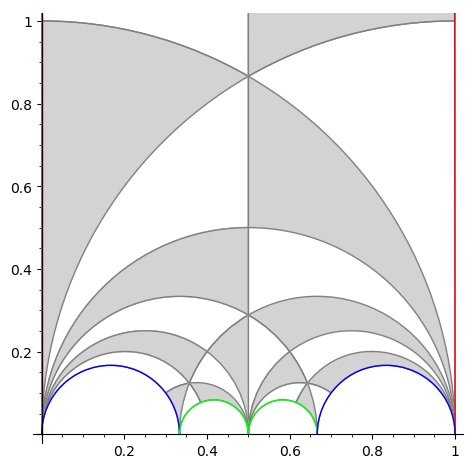But, there are plenty of other, seemingly unrelated, topics where $\Psi(n)$ appears. To name just a few:

• The number of points on the projective line $\mathbb{P}^1(\mathbb{Z}/n\mathbb{Z})$.
• The number of lattices at hyperdistance $n$ in Conway’s big picture.
• The number of admissible maximal commuting sets of operators in the Pauli group for the $n$ qudit.

and there are explicit natural one-to-one correspondences between all these manifestations of $\Psi(n)$, tbc.

Yesterday, there was an interesting post by John Baez at the n-category cafe: The Riemann Hypothesis Says 5040 is the Last.

The 5040 in the title refers to the largest known counterexample to a bound for the sum-of-divisors function
$\sigma(n) = \sum_{d | n} d = n \sum_{d | n} \frac{1}{n}$

In 1983, the french mathematician Guy Robin proved that the Riemann hypothesis is equivalent to
$\frac{\sigma(n)}{n~log(log(n))} < e^{\gamma} = 1.78107...$ when $n > 5040$.

The other known counterexamples to this bound are the numbers 3,4,5,6,8,9,10,12,16,18,20,24,30,36,48,60,72,84,120,180,240,360,720,840,2520.In Baez’ post there is a nice graph of this function made by Nicolas Tessore, with 5040 indicated with a grey line towards the right and the other counterexamples jumping over the bound 1.78107…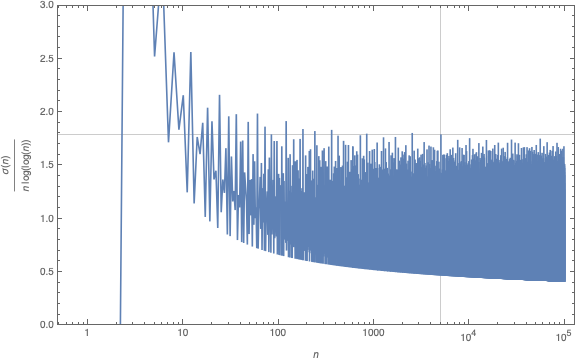Robin’s theorem has a remarkable history, starting in 1915 with good old Ramanujan writing a part of this thesis on “highly composite numbers” (numbers divisible by high powers of primes).

His PhD. adviser Hardy liked his result but called them “in the backwaters of mathematics” and most of it was not published at the time of Ramanujan’s degree ceremony in 1916, due to paper shortage in WW1.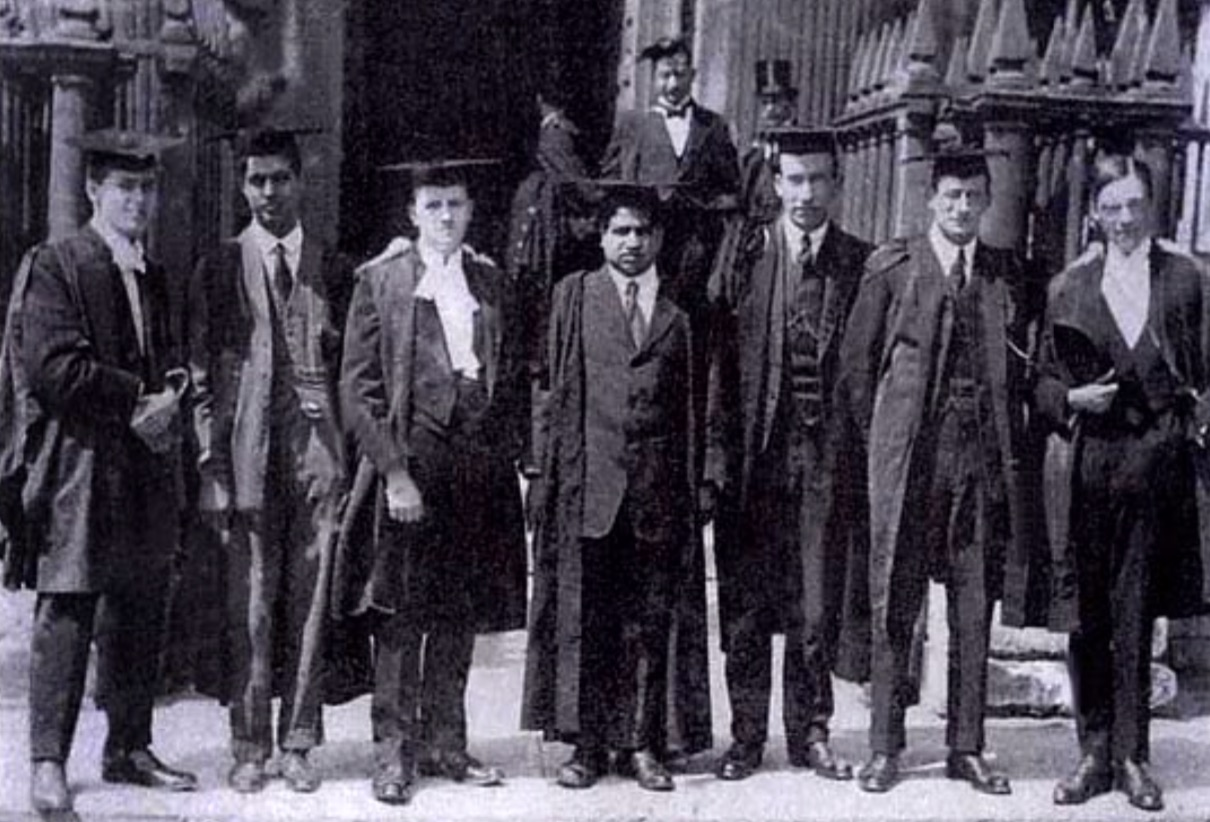When Ramanujan’s paper “Highly Composite Numbers” was first published in 1988 in ‘The lost notebook and other unpublished papers’ it became clear that Ramanujan had already part of Robin’s theorem.

Ramanujan states that if the Riemann hypothesis is true, then for $n_0$ large enough we must have for all $n > n_0$ that
$\frac{\sigma(n)}{n~log(log(n))} < e^{\gamma} = 1.78107...$ When Jean-Louis Nicolas, Robin's PhD. adviser, read Ramanujan's lost notes he noticed that there was a sign error in Ramanujan's formula which prevented him from seeing Robin's theorem.

Nicolas: “Soon after discovering the hidden part, I read it and saw the difference between Ramanujan’s result and Robin’s one. Of course, I would have bet that the error was in Robin’s paper, but after recalculating it several times and asking Robin to check, it turned out that there was an error of sign in what Ramanujan had written.”

If you are interested in the full story, read the paper by Jean-Louis Nicolas and Jonathan Sondow: Ramanujan, Robin, Highly Composite Numbers, and the Riemann Hypothesis.

What’s the latest on Robin’s inequality? An arXiv-search for Robin’s inequality shows a flurry of activity.

For starters, it has been verified for all numbers smaller that $10^{10^{13}}$…

It has been verified, unconditionally, for certain classes of numbers:

• all odd integers $> 9$
• all numbers not divisible by a 25-th power of a prime

Rings a bell? Here’s another hint:

According to Xiaolong Wu in A better method than t-free for Robin’s hypothesis one can replace the condition of ‘not divisible by an N-th power of a prime’ by ‘not divisible by an N-th power of 2’.

Further, he claims to have an (as yet unpublished) argument that Robin’s inequality holds for all numbers not divisible by $2^{42}$.

So, where should we look for counterexamples to the Riemann hypothesis?

What about the orders of huge simple groups?

The order of the Monster group is too small to be a counterexample (yet, it is divisible by $2^{46}$).

The final part, starting with a reaction of Mochizuki himself on my previous IUTeich-posts.

July 7th, 2013

Mochizuki update

While i’ve been away from G+, someone emailed Mochizuki my last post on the problem i have with Frobenioids1. He was kind enough to forward  me M’s reply:

“There is absolutely nothing difficult or subtle going on here (e.g., by comparison to the portion of the theory cited in the discussion preceding the statement of this “problem”!).  The nontrivial result is the fact that the degree is rational, i.e., the initial portion of [FrdI], Theorem 6.4, (iii), which is a consequence of a highly nontrivial result in transcendence theory due to Lang (i.e., [FrdI], Lemma 6.5, (ii)).  Once one knows this rationality, the conclusion that distinct prime numbers are not confused with one another is a formal consequence (i.e., no complicated subtle arguments!) of the fact that the ratio of the natural logarithm of any two distinct prime numbers is never rational.
Sincerely, Shinichi Mochizuki”

Being back, i’ll give it another go.

Having read the shared article below (2/5/2019 ‘the paradox of the proof’), it’s comforting to know that other people, including  +Aise Johan de Jong and +Cathy O’Neil, are also frustrated by M’s opaque latest writings.

July 11th, 2013

Mochizuki’s Frobenioid reconstruction: the final bit

In “The geometry of Frobenioids 1” Mochizuki ‘dismantles’ arithmetic schemes and replaces them by huge categories called Frobenioids. Clearly, one then wants to reconstruct the schemes from these categories and we almost understood how he manages to to this, modulo the ‘problem’ that there might be auto-equivalences of $Frob(\mathbb{Z})$, the Frobenioid corresponding to $\mathbf{Spec}(\mathbb{Z})$ i.e.the collection of all prime numbers, reshuffling distinct prime numbers.

In previous posts i’ve simplified things a lot, leaving out the ‘Arakelov’ information contained in the infinite primes, and feared that this lost info might be crucial to understand the final bit. Mochizuki’s email also points in that direction.

Here’s what i hope to have learned this week:

the full Frobenioid $Frob(\mathbb{Z})$

objects of $Frob(\mathbb{Z})$ consist of pairs $(q,r)$ where $q$ is a strictly positive rational number and $r$ is a real number.

morphisms are of the form $f=(n,a,(z,s)) : (q,r) \rightarrow (q’,r’)$ (where $n$ and $z$ are strictly positive integers, $a$ a strictly positive rational number and $s$ a positive real number) subject to the relations that

$q^n.z = q’.a \quad \text{and} \quad n.r+s = r’+log(a)$

$n$ is called the Frobenious degree of $f$ and $(z,s)$ the divisor of $f$.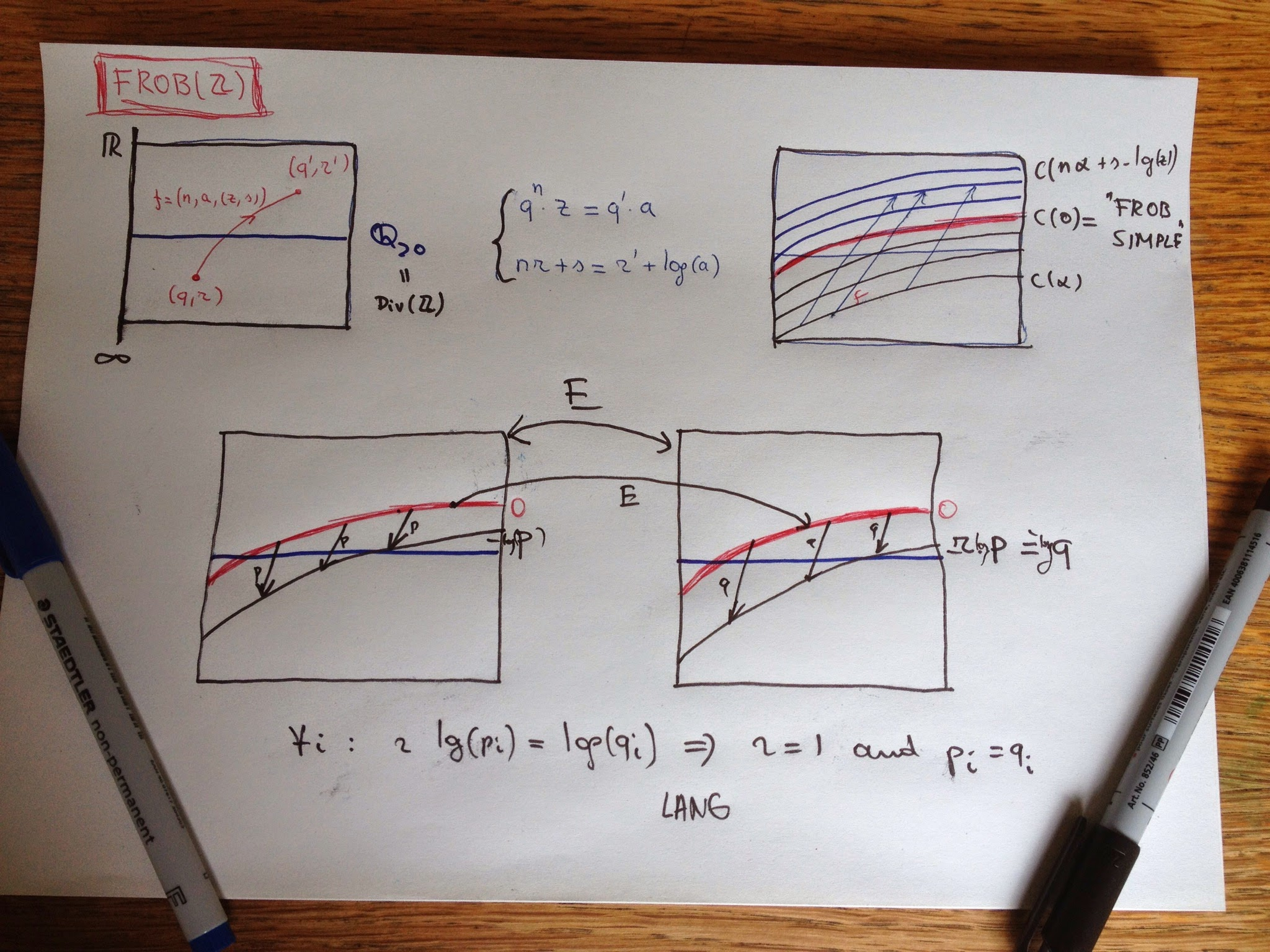All this may look horribly complicated until you realise that the isomorphism classes in $Frob(\mathbb{Z})$ are exactly the ‘curves’ $C(\alpha)$ consisting of all pairs $(q,r)$ such that $r-log(q)=\alpha$, and that morphisms with the same parameters as $f$ send points in $C(\alpha)$ to points in $C(\beta)$ where

$\beta = n.\alpha + s – log(a)$

So, the isomorphism classes can be identified with the real numbers $\mathbb{R}$ and special linear morphisms of type $(1,a,(1,s))$ and their compositions are compatible with the order-structure and addition on $\mathbb{R}$.

Crucial is Mochizuki’s observation that $C(0)$ are precisely the ‘Frobenious-trivial’ objects in $Frob(\mathbb{Z})$ (i’ll spare you the details but it is a property on having sufficiently many nice endomorphisms).

Now, consider an auto-equivalence $E$ of $Frob(\mathbb{Z})$. It will induce a map between the isoclasses $E : \mathbb{R} \rightarrow \mathbb{R}$ which is additive and as Frob-trivs are mapped under $E$ to Frob-trivs this will map $0$ to $0$, so $E$ will be an additive group-endomorphism on $\mathbb{R}$ hence of the form $x \rightarrow r.x$ for some fixed real number $r$, and we want to show that $r=1$.

Linear irreducible morphisms are of type $(1,a,(p,0))$ where $p$ is a prime number and they map $C(\alpha)$ to $C(\alpha-log(p))$. As irreducibles are preserved under equivalence this means that for each prime $p$ there must exist a prime $q$ such that $r log(p) = log(q)$.

Now if $r$ is an irrational number, there must be at least three triples $(p_1,q_1),(p_2,q_2)$ and $(p_3,q_3)$ satisfying $r log(p_i) = log(q_i)$ but this contradicts a fairly hard result, due to Lang, that for 6 distinct primes l_1,…,l_6 there do not exist positive rational numbers $a,b$ such that

$log(l_1)/log(l_2) = a log(l_3)/log(l_4) = b log(l_5)/log(l_6)$

So, $r$ must be rational and of the form $n/m$, but then $r=1$ (if not the correspondence $r.log(p) = log(q)$ gives $p^n=q^m$ contradicting unique factorisation). This then shows that under the auto-equivalence each prime $p$ (corresponding to a linear irreducible map) is send to itself.

This was the remaining bit left to show that the Frobenioid corresponding to any Galois extension of the rationals contains enough information to reconstruct from it the schemes of all rings of integers in intermediate fields.

August 15th, 2013

For travellers into Mochizuki-territory the indispensable rough guide is Lucien Szpiro’s ‘Marabout-Flash de théorie des nombres algébriques’, aka section I.1.3 in  ‘Séminaire sur les pinceaux arithmétiques: la conjecture de Mordell’.

Marabout Flash was a Franco-Belge series of do-it-yourself booklets, quite popular in the 60ties and 70ties, on almost every aspect of everyday’s life.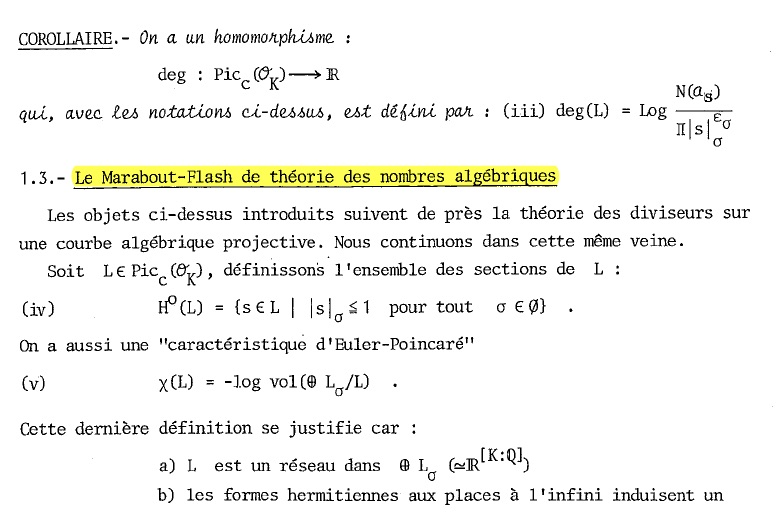In just a couple of pages Szpiro describes how one can extend the structure sheaf of a fractional ideal of a ring of integers to a ‘metrized’ (or Arakelov) line-bundle on the completed prime spectrum (including the infinite places). These bundles then satisfy properties similar to those of line-bundles on smooth projective curves, including a version of the Riemann-Roch theorem and a criterium to have non-zero global sections.

These (fairly simple) results then quickly lead to proofs of the first major results in number theory such as the Hermite-Minkowski theorem and Dirichlet’s unit theorem.

December 29th, 2014

Mochizuki in denial

From M’s 2014 IUTeich-Progress-Report (17 pages, the 2013-edition was only 7 pages long):

“Activities surrounding IUTeich appears to be in a stage of transition from a focus on verification to a focus on dissemination.”

If only…

He further lists hypotheses as to why nobody (apart from his 3 disciples Yamashita,Saidi and Hoshi) makes a serious effort to “study the theory carefully and systematically from the beginning”:

1. it is too long (1500-2500 pages)
2. there’s lack of textbooks on anabelian geometry
3. we are obsessed with the Langlands program
4. there’s little room for generalisations
5. it may not be directly useful for our own research

But then, why should anyone make such an effort, as:

“With the exception of the handful of researchers already involved in the verification activities concerning IUTeich, every researcher in arithmetic geometry throughout the world is a complete novice with respect to the mathematics surrounding IUTeich, and hence, in particular, is simply not qualified to issue a definite judgment concerning the validity of IUTeich on the basis of a ‘deep understanding’ arising from his/her previous research achievements.”

No Mochizuki, the next phase will not be dissemination, it will be denial.

Other remarkable sentences are:

“IUTeich is ‘the correct theory’ in the sense that it leads one to doubt the existence of any sort of ‘alternative proof’, i.e. via essentially different techniques, of the ABC Conjecture.”

and:

“the status of IUTeich in the field of arithmetic geometry constitutes a sort of faithful miniature model of the status of pure mathematics in human society.”

Already looking forward to the 2015 ‘progress’ report…

October 8th, 2015

Proud to be working at a well-known university

First time I’m mentioned in “Nature”, they issue this correction:

Corrected: An earlier version of this story incorrectly located the University of Antwerp in the Netherlands. It is in Belgium. The text has been updated.

Not particularly proud of the quote they took from my blog though:

“Is it just me, or is Mochizuki really sticking up his middle finger to the mathematical community”.

December 17th, 2017

Yesterday, my feeds became congested by (Japanese) news saying that Mochizuki’s (claimed) proof of the abc-conjecture had been vetted and considered fit to be published in a respectable journal.

Today, long term supporters of M’s case began their Echternachian-retreat after finding out that Mochizuki himself is the editor in charge of that respectable journal.

Attached is Ed Frenkel’s retraction of his previous tweet. Also, Taylor Dupuy deemed the latest action a bridge too far, see here.

Peter Woit did a great job in his recent post
pinpointing a critical argument in the 500-page long papers having as its “proof” that it followed trivially from the definitions…

I’d expect any board of editors to resign in such a case.
I fear this can only end badly.

Here’s batch 2 of my old google+ posts on ‘Inter Universal Teichmuller theory’, or rather on the number theoretic examples of Frobenioids.

June 5th, 2013

Mochizuki’s categorical prime number sieve

And now for the interesting part of Frobenioids1: after replacing a bunch of arithmetic schemes and maps between them by a huge category, we will reconstruct this classical picture by purely categorical means.

Let’s start with the simplest case, that of the ‘baby arithmetic Frobenioid’ dismantling $\mathbf{Spec}(\mathbb{Z}) (that is, the collection of all prime numbers) and replacing it by the category having as its objects all$(a)$where$a$is a strictly positive rational number and morphisms labeled by triples$(n,r,z)$where$n$and$z$are strictly positive integers and$r$is a strictly positive rational number and connecting two objects $(n,r,z) : (a) \rightarrow (b) \quad \text{ if and only if } \quad a^n.z=b.r$ Composition of morphisms is well-defined and looks like$(m,s,v) \circ (n,r,u) = (m.n,r^m.s,u^m.v)$as one quickly checks. The challenge is to recover all prime numbers back from this ‘Frobenioid’. We would like to take an object$(a)$and consider the maps$(1,1,p)$from it for all prime numbers$p$, but cannot do this as categorically we have to drop all labels of objects and arrows. That is, we have to recognize the map$(1,1,p)$among all maps starting from a given object.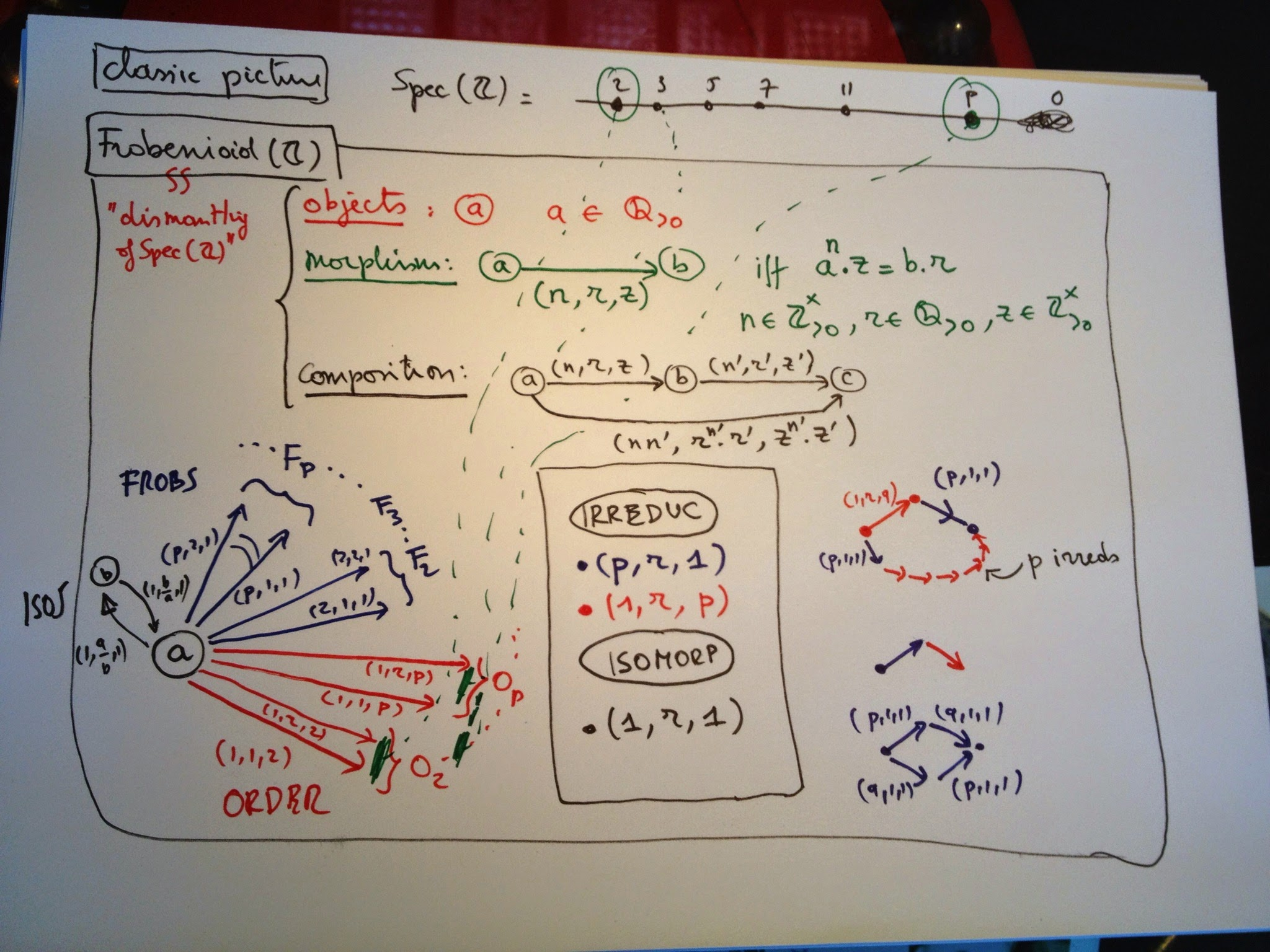We can identify all isomorphisms in the category and check that they are precisely the morphisms labeled$(1,r,1)$. In particular, this implies that all objects are isomorphic and that there is a natural correspondence between arrows leaving$(a)$and arrows leaving$(b)$by composing them with the iso$(1,b/a,1) : (b) \rightarrow (a)$. Another class of arrows we can spot categorically are the ‘irreducibles’, which are maps$f$which are not isos but have the property that in any factorization$f=g \circ h$either$g$or$h$must be an iso. One easily verifies from the composition rule that these come in two flavours: – those of Frobenius type :$(p,r,1)$for any prime number$p$– those of Order type :$(1,r,p)$for any prime number$p$We would like to color the froBs Blue and the oRders Red, but there seems to be no way to differentiate between the two classes by purely categorical means, until you spot Mochizuki’s clever little trick. start with a Red say$(1,r,q)$for a prime number$q$and compose it with the Blue$(p,1,1)$, then you get the morphism$(p,r^p,q^p)$which you can factor as a composition of$p+1$irreducibles $(p,r^p,q^p) = (1,r,q) \circ (1,r,q) \circ …. \circ (1,r,q)o(p,1,1)$ and if$p$grows, so will the number of factors in this composition. On the other hand, if you start with a Blue and compose it with either a Red or a Blue irreducible, the obtained map cannot be factored in more irreducibles. Thus, we can identify the Order-type morphisms as those irreducibles$f$for which there exists an irreducible$g$such that the composition$g \circ f$can be factored as the composition in at least$n$irreducibles, where we can take n arbitrarily large. Finally we say that two Reds out of$(a)$are equivalent iff one is obtained from the other by composing with an isomorphism and it is clear that the equivalence classes are exactly the arrows labeled$(1,r,p)$for fixed prime number$p$. So we do indeed recover all prime numbers from the category. Similarly, we can see that equivalence classes of Frobs from$(a)$are of the form$(p,r,1)$for fixed prime$p$. An amusing fact is that we can recover the prime$p$for a Frob by purely categorical ways using the above long factorization of a composition with a Red. There seems to be no categorical way to determine the prime number associated to an equivalence class of Order-morphisms though… Or, am i missing something trivial? June 7th, 2013 Mochizuki’s Frobenioids for the Working Category Theorist Many of you, including +David Roberts +Charles Wells +John Baez (and possibly others, i didn’t look at all comments left on all reshares of the past 4 posts in this MinuteMochizuki project) hoped that there might be a more elegant category theoretic description of Frobenioids, the buzz-word apparently being ‘Grothendieck fibration’ … Hence this attempt to deconstruct Frobenioids. Two caveats though: – i am not a category theorist (the few who know me IRL are by now ROFL) – these categories are meant to include all arithmetic information of number fields, which is a messy business, so one should only expect clear cut fibrations in easy situation such as principal ideal domains (think of the integers$\mathbb{Z}$). Okay, we will try to construct the Frobenioid associated to a number field$K$(that is, a finite dimensional extension of the rationals$\mathbb{Q}$) with ring of integers$R$(the integral closure of$\mathbb{Z}$in$K$). For a concrete situation, look at the quadratic case. The objects will be fractional ideals of K which are just the R-submodules$I$of$K$such that there in an$r$in$R$such that$I.r$is a proper ideal of$R$. Dedekind showed that any such thing can be written uniquely as a product $I = P_1^{a_1} … P_k^{a_k}$ where the$P_i$are prime ideals of$R$and the$a_i$are integers (if they are all natural numbers, I will be a proper ideal of$R$). Clearly, if one multiplies two fractional ideals$I$and$J$, the result$I.J$is again a fractional ideal, so they form a group and by Dedekind’s trick this group is the free Abelian group on all prime ideals of$R$.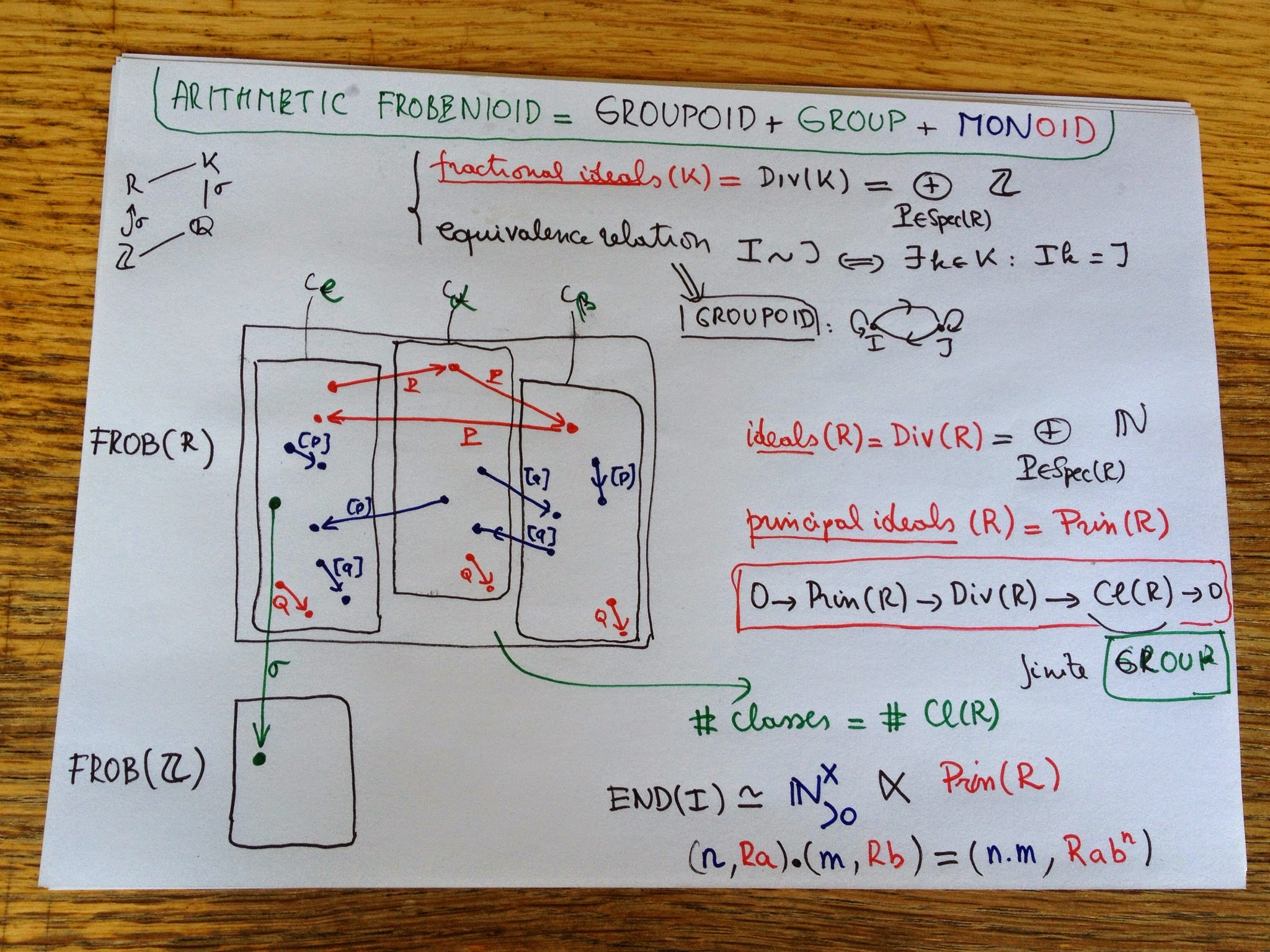Next, we define an equivalence relation on this set, calling two fractional ideals$I$and$J$equivalent if there is a$k$in$K$such that$I=J.k$(or if you prefer, if they are isomorphic as$R$-modules). We have a set with an equivalence relation and hence a groupoid where these is a unique isomorphism between any two equivalent objects. This groupoid is precisely the groupoid of isomorphisms of the Frobenioid we’re after. The number of equivalence classes is finite and these classes correspond to the element of a finite group$Cl(R)$called the class group of$R$which is the quotient group of ideals modulo principal ideals (so if your$R$is a principal ideal domain there is just one component). The ‘groups’ corresponding to each connected component of the groupoid are all isomorphic to the quotient group of the units in$K$by the units in$R$. Next, we will add the other morphisms. By definition they are all compositions of irreducibles which come in 2 flavours: – the order-morphisms$P$for any prime ideal$P$of$R$sending$I$to$I.P$. Typically, these maps switch between different equivalence classes (unless$P$itself is principal). We can even explicitly compute small norm prime ideals which will generate all elements in the class group$Cl(R)$. – the power-maps$[p]$for any prime number$p$which sends$I$to$I^p$. The nature of these maps really depend on the order of the component in the finite group$Cl(R)$. Well, that’s it basically for the layer of the Frobenioid corresponding to the number field$K$. (You have to repeat all this for any subfield between$\mathbb{Q}$and$K$). A cute fact is that all endomorphism-monoids of objects in the layer of K are all isomorphic as abstract monoid to the skew-monoid$\mathbb{N}^x_{>0} x Prin(R)$of the multiplicative group of all strictly positive integers$n$with the monoid of all principal ideals in R with multiplication defined by $(n,Ra).(m,Rb)=(nm,Rab^n)$ The only extra-type morphisms we still have to include are those between the different layers of the Frobenioid, the green ones which M calls the pull-back morphisms. They are of the following form: if$R_1$and$R_2$are rings of integers in the fields$K_1$contained in$K_2$, then for any ringmorphism$\sigma : R_1 \rightarrow R_2$one can extend a fractional ideal$I$of$R_1$to$K_2$by considering$R_2.\sigma(I)$. These then give the morphism$r_2\sigma(I) \rightarrow I$and as we will see in a next instalment, they encode the splitting behaviour of prime ideals. a question for category people Take the simplest situation, that of the integers$\mathbb{Z}$. So, we have just a groupoid with extra morphisms generated by the order-maps$o_p$and the power maps$f_p$. The endo-ring of any object is then isomorphic top the abstract group generated by the$f_p$and$o_p$and satisfying following relations $o_p.o_q=o_q.o_p$ $f_p.f_q=f_q.f_p$ $f_p.o_q=o_q^p.f_p$ My question now is: if for two different primes$p$and$q$i switch their role in the endo-ring of 1 object and propagate this via all isos to all morphisms, do i get a category equivalence? (or am i missing something?). (tbc) June 11th, 2013 my problem with Mochizuki’s Frobenioid1 Let us see how much arithmetic information can be reconstructed from an arithmetic Frobenioids. Recall that for a fixed finite Galois extension$L$of$\mathbb{Q}$this is a category with objects all fractional ideals in subfields of$L$, and maps generated by multiplication-maps with ideals in rings of integers, power-maps and Galois-extension maps. When all objects and morphisms are labeled it is quite easy to reconstruct the Galois field$L$from it as well as all maps between prime spectra of rings of integers in intermediate fields, which after all was the intended use of Frobenioids, to ‘dismantle’ these arithmetic schemes and endow them with extra structure given by the power-maps.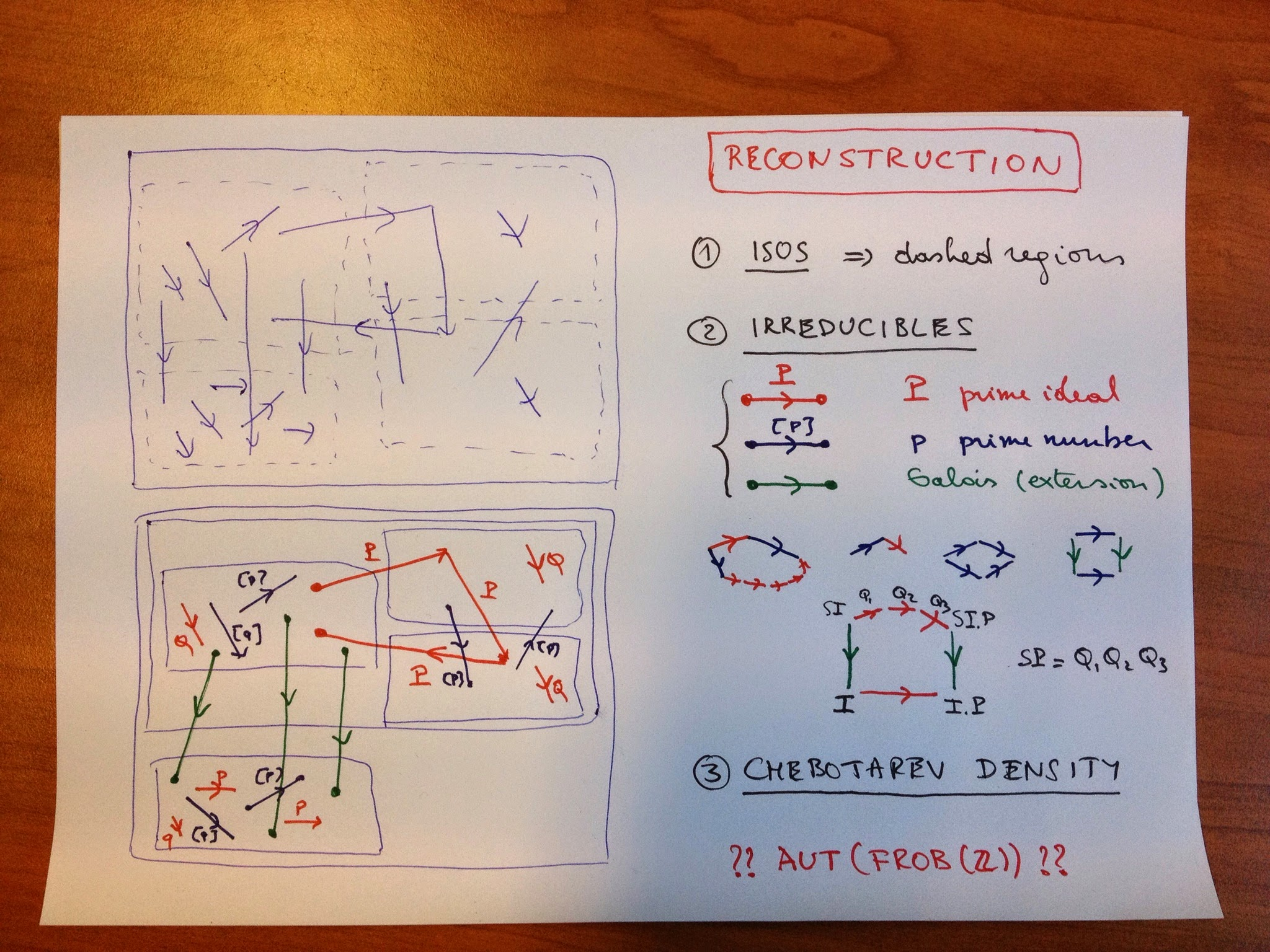However, in this reconstruction process we are only allowed t use the category structure, so all objects and morphisms are unlabelled (the situation top left) and we want to reconstruct from it the different layers of the Frobenioid (corresponding to the different subfields) and divide all arrows according to their type (situation bottom left). First we can look at all isomorphisms. They will divide the category in the dashed regions, some of them will be an entire layer (for example for$\mathbb{Q}$) but in general a finite number of these regions will make up the full layer of a subfield (the regions labeled by the elements of the ideal class group). Another categorical notion we can use are ‘irreducible morphisms’, that is a morphism$f$which is not an iso but having the property that in each factorisation$f = g \circ h$either$g$or$h$must be an iso. If we remember the different types of morphisms in our Frobenioid we see that the irreducibles come in 3 flavours: – oRder-maps (Red) : multiplication by a prime ideal$P$of the ring of integers of the subfield – froBenius or power-maps (Blue) sendingg a fractional ideal$I$to$I^p$for a prime number$p$– Galois-maps (Green) extending ideals for a subfield$K$to$K’$having no intermediate field. We would like to determine the colour of these irreducibles purely categorical. The idea is that reds have the property that they can be composed with another irreducible (in fact, of power type) such that the composition can again be decomposed in irreducibles and that there is no a priori bound on the number of these terms (this uses the fact that$[p] \circ Q = Q \circ Q \circ … Q \circ [p]$and that there are infinitely many prime numbers$p$). One checks that compositions of order or Galois maps with irreducibles have factorisation with a bounded number of irreducibles. The most interesting case is the composition of a Galois map with an order map$P$, this can be decomposed alternatively as order maps in the bigger field followed by a Galois map, the required order maps are the bigger primes$Q_i$occurring in the decomposition of the extended ideal $S.P = Q_1.Q_2….Q_k$ but the number$k$of this decomposition is bounded by the dimension of the bigger field over the smaller one. Summarizing: – we can determine all the red maps, which will then give us also the different layers – we can determine the green maps as they move between different layers – to the remaining blue ones we can even associate their label$[p]$by the observed property of composition with order maps. Taking an object in a layer, we get the set of prime ideals of the ring on integers in that field as the set of all red arrows leaving that object unto equivalence (by composing with an isomorphism), so we get the prime spectra$\mathbf{Spec}(S)$. For a ring-extension$R \rightarrow S$we also can recover the cover map$\mathbf{Spec}(S) \rightarrow \mathbf{Spec}(R)$Indeed, composing the composition of the Galois map with the order-map$P$and decomposing it alternatively will give us the finite number of prime ideals$Q_i$of$S$lying over$P$. That is, we get all splitting behaviour of prime ideals in intermediate field-extensions. Let$K$be a Galois subfield of$L$then we have a way to see how a prime ideals in$\mathbf{Spec}(\mathbb{Z})$splits, ramifies or remains inert in$K$and so by Chebotarev density this gives us the dimension of$K$as well as the Galois group. And, if we could label the prime ideal by a prime number$p$, we could even reconstruct$K$itself as$K$is determined once we know all prime numbers which completely split. The problem i have is that i do not see a categorical way to label the red arrows in$Frob(\mathbb{Z})$by prime numbers. Mochizuki says we can do this in the proof of Thm 6.4(iii) by using the fact that the$log(p)$are linearly independent over$\mathbb{Q}$. This suggests that one might use the ‘Arakelov information’ (that is the archimidean valuations) to do this (the bit i left out so far), but i do not see this in the case of$\mathbb{Q}\$ as there is just 1 extra (real) valuation determined by the values of the nonarchimidean valuations.

Probably i am missing something so all sorts of enlightenment re welcome!